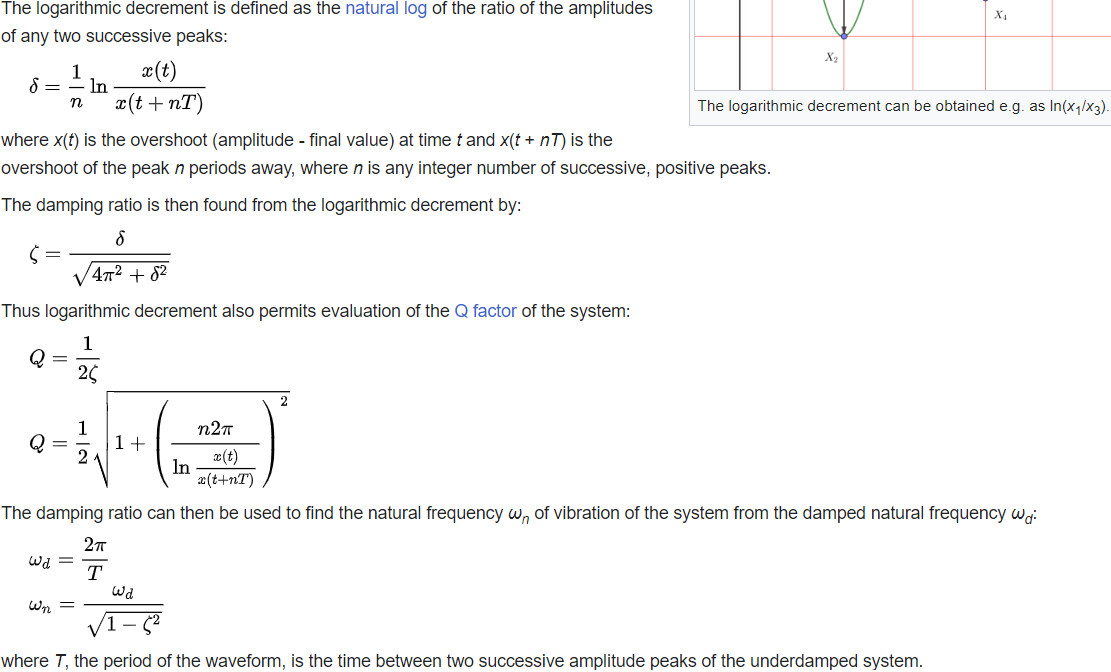# For loop variable error

2 views (last 30 days)
AL on 16 Mar 2023
Commented: AL on 16 Mar 2023
Mass Matrix
Motor_plate = 17.010;
First_Story = 2.162;
Sec_Story = 2.162;
Top_strory = 1.790;
L_leg = 0.278;
S_Leg = 0.042;
bracket =19.75/1000;
boltnut =12.45/1000 ;
%Unbalance_mass = 0.044;
m1 = Motor_plate + 4*L_leg + 4*S_Leg + First_Story+40*bracket+64*boltnut;
m2 = 4*S_Leg+ First_Story+16*bracket+16*bracket;
m_for_k = [m1 m2];
M1 = Motor_plate + 2*S_Leg +2*L_leg ; %In kgs
M2 = First_Story+ 4*S_Leg;
M3 = Sec_Story + 4 * S_Leg;
M4 = Top_strory+2*S_Leg;
V = [M1 M2 M3 M4];
M = diag(V); % in Kgs
clear M1 M2 M3 M4 m1 m2 Motor_plate First_Story Sec_Story S_Leg;
clear Top_strory V L_leg bracket boltnut
% preallocate output variables
n_files = 2;
Time = cell(n_files, 1);
Veloc = cell(n_files, 1);
Ypk_plot = cell(n_files, 1);
Xpk_plot = cell(n_files, 1);
Y = cell(n_files, 1);
X = cell(n_files, 1);
P = cell(n_files, 1);
damp_ratio = zeros(1, n_files);
wd = zeros(1, n_files);
wn = zeros(1, n_files);
K_ex = zeros(1, n_files);
% read data from files and process each file
for i = 1:n_files
z1 = importdata("Xxsv0000"+num2str(i)+".txt");
% extract time and velocity data
Time{i} = z1.data(:,1);
Veloc{i} = z1.data(:,2);
% find local maxima in velocity data within the range of indices
[Ypk, Xpk] = findpeaks(Veloc{i}(1:550));
Ypk_plot{i} = Ypk(11:35);
Xpk_plot{i} = Xpk(11:35);
Y{i} = Ypk;
X{i} = Xpk;
P{i} = zeros(size(Ypk));
% plot data against time data and mark the locations of the peaks found
figure(i)
plot(Time{i}, Veloc{i}, 'DisplayName', 'data')
hold on
plot(Time{i}(Xpk_plot{i}), Ypk_plot{i}, 'dr', 'DisplayName', 'Pick points')
grid on
legend('show')
sgtitle(['File ', num2str(i)])
% calculate logarithmic decrement, damping ratio, and natural frequency
t_new = Time{i}(X{i});
y_new = Y{i};
Log_Dec = abs(log(y_new(1:end-1) ./ y_new(2:end)));
Mean_dec = mean(Log_Dec);
Damp_period = (t_new(end) - t_new(1)) / length(t_new);
wd(i) = 2*pi / Damp_period;
damp_ratio(i) = Mean_dec / sqrt(1 + Mean_dec^2);
wn(i) = wd(i) * sqrt(1 - damp_ratio(i)^2);
% calculate spring constant
K_ex(i) = m_for_k * wn(i)^2;
end
--------------------------------------------------------------------------------------------------------------------------------------------------------------------------I am facing error with K_ex(i). Am i going in right direction or making any mistake?
Kindly help me.

Walter Roberson on 16 Mar 2023
m_for_k = [m1 m2];
vector.
K_ex(i) = m_for_k * wn(i)^2;
vector times scalar gives vector. Vector cannot be stored in a scalar location.
AL on 16 Mar 2023
Thank you so much.

### Categories

Find more on Loops and Conditional Statements in Help Center and File Exchange

R2022b

### Community Treasure Hunt

Find the treasures in MATLAB Central and discover how the community can help you!

Start Hunting!